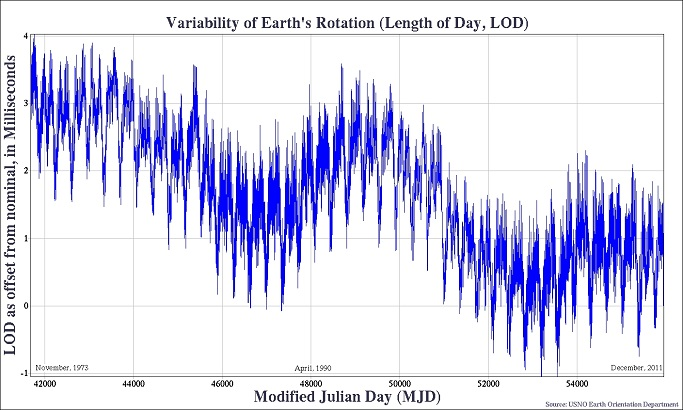# Systems of Time

"Now let me see," the Golux said. "If you can touch the clocks and never start them, then you can start the clocks and never touch them. That's logic, as I know and use it...."
-- James Thurber, "The 13 Clocks"

• Atomic Time, with the unit of duration the Systeme International (SI) second defined as the duration of 9,192,631,770 cycles of radiation corresponding to the transition between two hyperfine levels of the ground state of cesium 133. TAI is the International Atomic Time scale, a statistical timescale based on a large number of atomic clocks.

• Universal Time (UT) is counted from 0 hours at midnight, with unit of duration the mean solar day, defined to be as uniform as possible despite variations in the rotation of the Earth.
• UT0 is the rotational time of a particular place of observation. It is observed as the diurnal motion of stars or extraterrestrial radio sources.
• UT1 is computed by correcting UT0 for the effect of polar motion on the longitude of the observing site. It varies from uniformity because of the irregularities in the Earth's rotation.
• Coordinated Universal Time (UTC) differs from TAI by an integral number of seconds. UTC is kept within 0.9 seconds of UT1 by the introduction of one-second steps to UTC, the "leap second." To date these steps have always been positive. UTC has replaced Greenwich Mean Time (GMT) as an international standard.

• Dynamical Time replaced ephemeris time as the independent argument in dynamical theories and ephemerides. Its unit of duration is based on the orbital motions of the Earth, Moon, and planets.
• Terrestrial Time (TT), (or Terrestrial Dynamical Time, TDT), with unit of duration 86400 SI seconds on the geoid, is the independent argument of apparent geocentric ephemerides. TDT = TAI + 32.184 seconds.
• Barycentric Dynamical Time (TDB), is the independent argument of ephemerides and dynamical theories that are referred to the solar system barycenter. TDB varies from TT only by periodic variations.
• Geocentric Coordinate Time (TCG) is a coordinate time having its spatial origin at the center of mass of the Earth. TCG differs from TT as: TCG - TT = Lg x (JD -2443144.5) x 86400 seconds, with Lg = 6.969291e-10.

• Barycentric Coordinate Time (TCB)is a coordinate time having its spatial origin at the solar system barycenter. TCB differs from TDB in rate. The two are related by: TCB - TDB = iLb x (JD -2443144.5) x 86400 seconds, with Lb = 1.550505e-08.

• Sidereal Time, with unit of duration the period of the Earth's rotation with respect to a point nearly fixed with respect to the stars, is the hour angle of the vernal equinox.
Delta T is the difference between Earth rotational time (UT1) and dynamical time (TDT). Predicted values of UT1 - UTC are provided as an Earth Orientation Product.  An example showing the variation of the length of the day to late 2011 is shown below. Units are milliseconds.Julian Day Number is a count of days elapsed since Greenwich mean noon on 1 January 4713 BCE, Julian proleptic calendar. The Julian Date is the Julian day number followed by the fraction of the day elapsed since the preceding noon.

We frequently make use of the Modified Julian Date (MJD), which is defined as MJD = JD - 2400000.5. An MJD day thus begins and ends at midnight. Julian dates can be expressed in UT, TAI, TDT, etc. and so for precise applications the timescale should be specified, e.g. MJD 49135.3824 TAI.